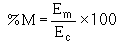Custom SearchANSWERS TO QUESTIONS Q1. THROUGH Q46. A1. Modulation is the impressing of intelligence on a transmission medium. A2. May be anything that transmits information, such as light, smoke, sound, wire lines, or radio-frequency waves. A3. Mixing two frequencies across a nonlinear impedance. A4. The process of recovering intelligence from a modulated carrier. A5. The sine wave. A6. To represent quantities that have both magnitude and direction. A7. Sine q = opposite side / hypotenuse. A8. e = Emax sine q. A9. The value at any given point on the sine wave. A10. Phase or phase angle. A11. The rate at which the vector which is generating the sine wave is rotating. A12. The elapsed time from the beginning of cycle to its completion. A13. Wavelength = rate of travel X period. A14. Process of combining two signal frequencies in a nonlinear device. A15. An impedance in which the resulting current is not proportional to the applied voltage. A16. The display of electromagnetic energy that is arranged according to wavelength or frequency. A17. At least two different frequencies applied to a nonlinear impedance. A18. Any method of modulating an electromagnetic carrier frequency by varying its amplitude in accordance with the intelligence. A19. A method of generating oscillations, a method of turning the oscillations on and off (keying), and an antenna to radiate the energy. A20. Plate keying and cathode keying. A21. Machine keying. A22. A high degree of clarity even under severe noise conditions, long-range operation, and narrow bandwidth. A23. Antenna-to-ground capacitance can cause the oscillator frequency to vary. A24. To isolate the oscillator from the antenna and increase the amplitude of the rf oscillations to the required output level. A25. To raise the low frequency of a stable oscillator to the vhf range. A26. An energy converter that changes sound energy into electrical energy. A27. The changing resistance of carbon granules as pressure is applied to them. A28. Background hiss resulting from random changes in the resistance between individual carbon granules. A29. The piezoelectric effect. A30. A dynamic microphone has a moving coil and the magnetic microphone has a moving armature. A31. Rf and af units. A32. 100 kilohertz, 5 kilohertz, 95 kilohertz, and 105 kilohertz. A33. All of the sum frequencies above the carrier. A34. The intelligence is contained in the spacing between the carrier and sideband frequencies. A35. The highest modulating frequency. A36. The depth or degree of modulation. A37. One-half the amplitude of the carrier. A38.A39. Modulation produced in the plate circuit of the last radio stage of the system. A40. Class C. A41. Power amplifier. A42. Between 0 and nearly two times its unmodulated value. A43. Plate modulator. A44. In cases when the use of a minimum of af modulator power is desired. A45. Low-level. A46. Gain is varied by changing the voltage on the emitter.Integrated Publishing, Inc. - A (SDVOSB) Service Disabled Veteran Owned Small Business